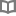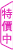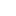當死者說話時 - 克拉斯・布許曼 - BOOKWALKER中文電子書

當死者說話時

犯罪迷必讀！最緊張懸疑的第一手真實案件，看資深法醫如何抽絲剝繭，為死者發聲

作者： 克拉斯・布許曼 譯者： 魏佐君

•克拉斯・布許曼 (Claas Buschmann)

一九七七年出生於漢堡。他曾在柏林夏里特醫學院（Charité）法醫部門擔任資深法醫多年，現於什勒斯維希-霍爾斯坦大學醫院法醫系擔任首席資深法醫。在司法機關的委託下，布許曼不僅在偵調兇殺案時予以醫學方面的幫助，也會協助釐清自殺或醫療事故的真相。私底下的布許曼則更為活潑：他曾用Dr. Boogie的名字在多場爵士音樂會上演奏鋼琴，除此之外，他還是AC/DC樂團的熱情粉絲。

• 放入購物車
•試閱

• 放入購物車
•試閱• 放入購物車
•試閱

• 放入購物車
•試閱

• 放入購物車
•無試閱

• 放入購物車
•試閱

• 放入購物車
•試閱

• 放入購物車
•試閱• 放入購物車
•試閱• 放入購物車
•試閱

• 放入購物車
•試閱

• 放入購物車
•試閱

• 放入購物車
•試閱

• 放入購物車
•試閱• 放入購物車
•試閱

• 放入購物車
•試閱

• 放入購物車
•試閱

• 放入購物車
•試閱

• 放入購物車
•試閱

• 放入購物車
•試閱

• 放入購物車
•試閱

• 放入購物車
•試閱

• 放入購物車
•試閱• 放入購物車
•試閱

• 放入購物車
•試閱

• 放入購物車
•試閱

• 放入購物車
•試閱

• 放入購物車
•試閱

• 放入購物車
•試閱

• 放入購物車
•試閱

• 放入購物車
•試閱

• 放入購物車
•試閱

• 放入購物車
•試閱

• 放入購物車
•試閱

• 放入購物車
•試閱

• 放入購物車
•試閱

• 放入購物車
•試閱

• 放入購物車
•試閱

• 放入購物車
•試閱

• 放入購物車
•試閱

• 放入購物車
•試閱• 放入購物車
•試閱

• 放入購物車
•試閱

• 放入購物車
•試閱